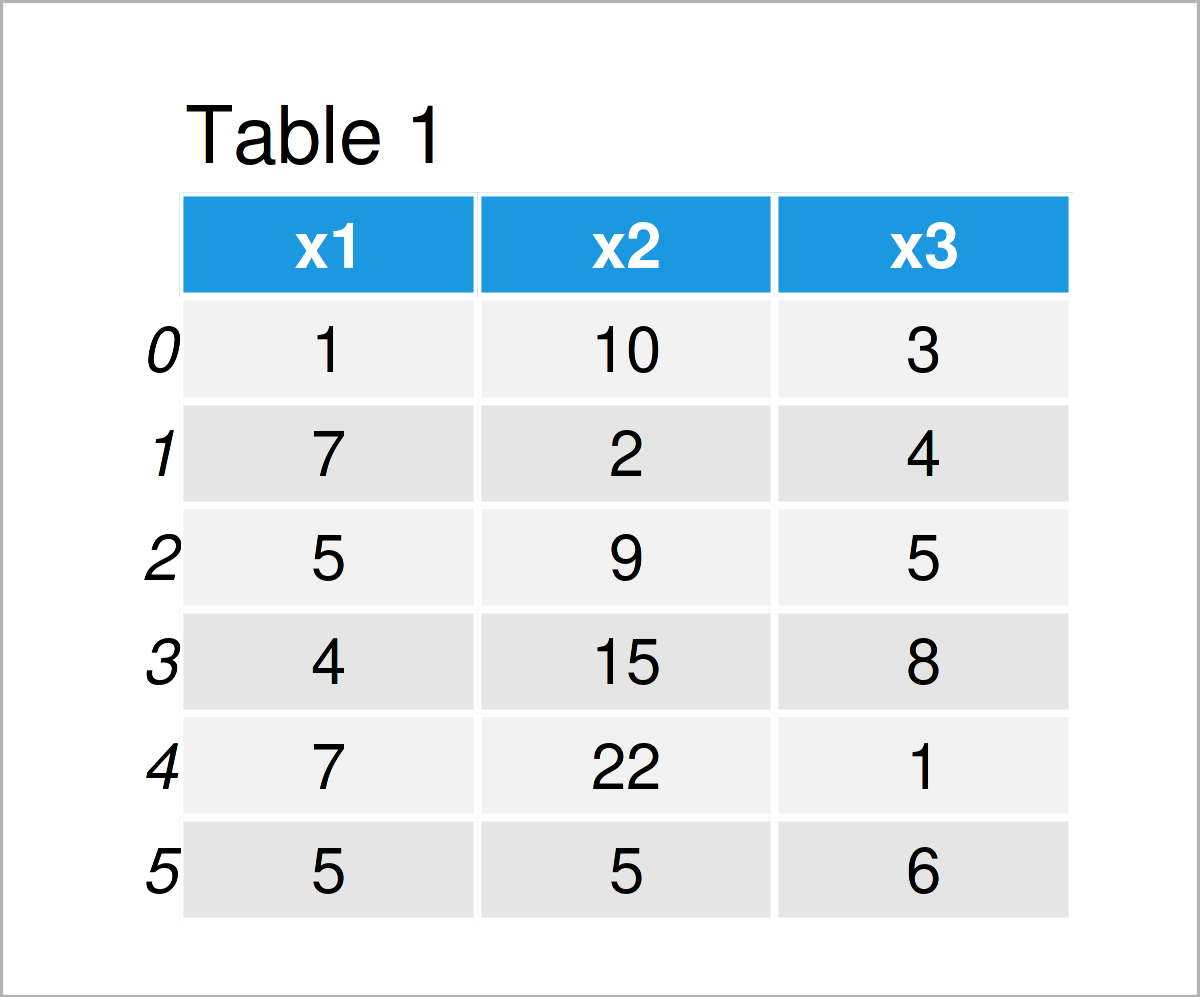# Convert String to Integer in pandas DataFrame Column in Python (4 Examples)

In this Python tutorial you’ll learn how to transform a pandas DataFrame column from string to integer.

So now the part you have been waiting for – the examples.

## Example Data & Libraries

First, we need to load the pandas library:

`import pandas as pd                                        # Import pandas`

The following data is used as a basis for this tutorial:

```data = pd.DataFrame({'x1':['1', '7', '5', '4', '7', '5'],  # Create pandas DataFrame
'x2':['10', '2', '9', '15', '22', '5'],
'x3':['3', '4', '5', '8', '1', '6']})
print(data)                                                # Print pandas DataFrame```Table 1 shows the structure of our example pandas DataFrame – It has six rows and three columns.

Let’s check the classes of the variables in our pandas DataFrame:

```print(data.dtypes)                                         # Check data types of columns
# x1    object
# x2    object
# x3    object
# dtype: object```

As you can see, each of the columns in our example data set has the object data type. Note that the pandas library uses the object dtype for storing strings, i.e. actually the columns in our example DataFrame are strings.

The following examples show different ways on how to convert pandas DataFrame columns to the integer class.

Let’s move on to the examples!

## Example 1: Convert Single pandas DataFrame Column from String to Integer

Example 1 demonstrates how to convert one specific pandas DataFrame column from string to the integer data type.

For this task, we can apply the astype function as shown below:

```data_new1 = data.copy()                                    # Create copy of DataFrame
data_new1['x1'] = data_new1['x1'].astype(int)              # Transform string to integer```

Let’s return the data types of our updated pandas DataFrame:

```print(data_new1.dtypes)                                    # Check data types of columns
# x1     int32
# x2    object
# x3    object
# dtype: object```

As you can see, we have converted the first column x1 to the integer class. The other columns still have the object (i.e. string) dtype.

## Example 2: Convert Multiple pandas DataFrame Columns from String to Integer

Example 2 illustrates how to transform multiple variables from the string data type to integer.

For this, we can apply the following Python syntax:

```data_new2 = data.copy()                                    # Create copy of DataFrame
data_new2 = data_new2.astype({'x2': int, 'x3': int})       # Transform multiple strings to integer```

Let’s check the data types once again:

```print(data_new2.dtypes)                                    # Check data types of columns
# x1    object
# x2     int32
# x3     int32
# dtype: object```

This time, we have switched the data classes of the columns x2 and x3 to the integer class.

## Example 3: Convert All pandas DataFrame Columns from String to Integer

It is also possible to change the data type of all columns simultaneously from string to integer.

Consider the Python code below:

```data_new3 = data.copy()                                    # Create copy of DataFrame
data_new3 = data_new3.astype(int)                          # Transform all columns to integer```

Now, we can have another look at the data types of the columns of our pandas DataFrame:

```print(data_new3.dtypes)                                    # Check data types of columns
# x1    int32
# x2    int32
# x3    int32
# dtype: object```

As you can see, all columns have been converted to the integer data type.

## Example 4: Convert pandas DataFrame Column from String to Integer Using to_numeric() Function

So far, we have only used the astype function to modify and adjust the classes of our pandas DataFrame columns.

This example, in contrast, demonstrates how to use the to_numeric function for this task:

```data_new4 = data.copy()                                    # Create copy of DataFrame
data_new4['x1'] = pd.to_numeric(data_new4['x1'])           # Apply to_numeric function```

Once again, let’s print the data types of all columns:

```print(data_new4.dtypes)                                    # Check data types of columns
# x1     int64
# x2    object
# x3    object
# dtype: object```

Similar to Example 1, we have changed the data type of the variable x1 from string to integer. However, this time we have used the to_numeric function instead of the astype function.

## Video & Further Resources

Have a look at the following video on my YouTube channel. I illustrate the Python codes of this article in the video.

Please accept YouTube cookies to play this video. By accepting you will be accessing content from YouTube, a service provided by an external third party.If you accept this notice, your choice will be saved and the page will refresh.

Besides that, you might read the related tutorials on this website.

This tutorial has illustrated how to convert and parse a pandas DataFrame column from string to integer in Python. Don’t hesitate to let me know in the comments, in case you have any further questions or comments. Furthermore, please subscribe to my email newsletter for updates on new articles.

Subscribe to the Statistics Globe Newsletter